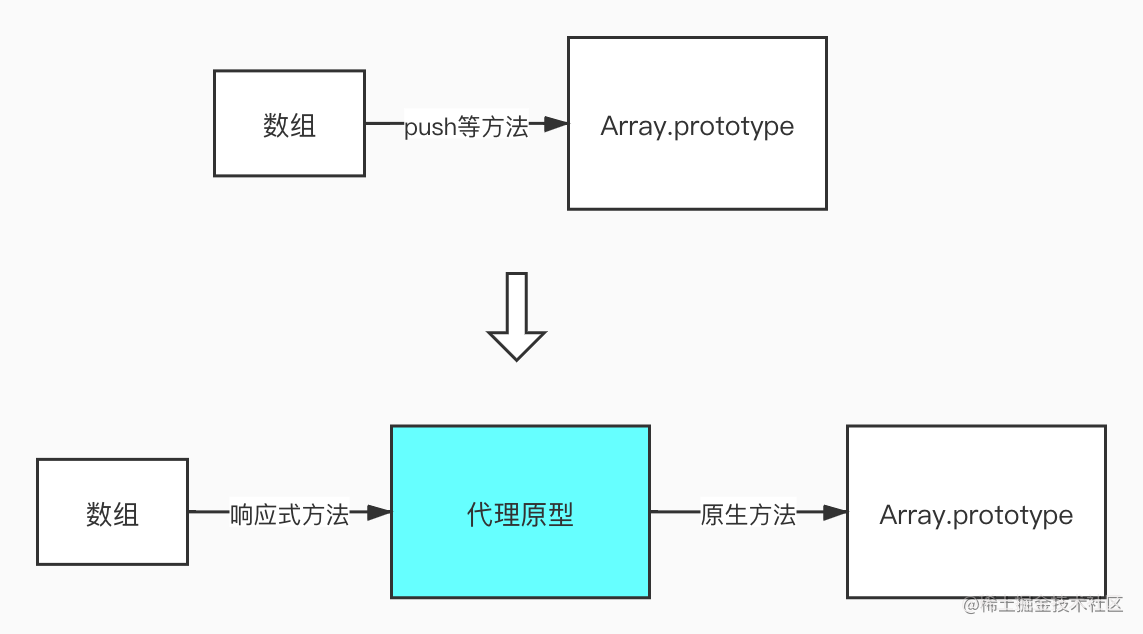# 0年前端的Vue响应式原理学习总结2：数组的处理

1：基本原理

3：渲染watcher

4：最终章

## 1. 为什么要对数组特殊处理

``````function defineReactive(obj, key, val) {
Object.defineProperty(obj, key, {
get() {
console.log('get: ', val)
return val
},
set(newVal) {
console.log('set: ', newVal)
newVal = val
}
})
}

const arr = [1, 2, 3]
arr.forEach((val, index, arr) => {
defineReactive(arr, index, val)
})

``````class Observer {
constructor(value) {
this.value = value
if (Array.isArray(value)) {
// 代理原型...
this.observeArray(value)
} else {
this.walk(value)
}
}

walk(obj) {
Object.keys(obj).forEach((key) => defineReactive(obj, key, obj[key]))
}
// 需要继续监听数组内的元素(如果数组元素是对象的话)
observeArray(arr) {
arr.forEach((i) => observe(i))
}
}

## 2. 代理原型``````// Observer.js
if (Array.isArray(value)) {
Object.setPrototypeOf(value, proxyPrototype) // value.__proto__ === proxyPrototype
this.observeArray(value)
}

// array.js
const arrayPrototype = Array.prototype // 缓存真实原型

// 需要处理的方法
const reactiveMethods = [
'push',
'pop',
'unshift',
'shift',
'splice',
'reverse',
'sort'
]

// 增加代理原型 proxyPrototype.__proto__ === arrayProrotype
const proxyPrototype = Object.create(arrayPrototype)

// 定义响应式方法
reactiveMethods.forEach((method) => {
const originalMethod = arrayPrototype[method]
// 在代理原型上定义变异响应式方法
Object.defineProperty(proxyPrototype, method, {
value: function reactiveMethod(...args) {
const result = originalMethod.apply(this, args) // 执行默认原型的方法
// ...派发更新...
return result
},
enumerable: false,
writable: true,
configurable: true
})
})

## 3. `__ob__`属性

`observe`做一个修改：

``````// observe.js
function observe(value) {
if (typeof value !== 'object') return
let ob
// __ob__还可以用来标识当前对象是否被监听过
if (value.__ob__ && value.__ob__ instanceof Observer) {
ob = value.__ob__
} else {
ob = new Observer(value)
}
return ob
}

`Observer`也要做修改：

``````constructor(value) {
this.value = value
this.dep = new Dep()
// 在每个对象身上定义一个__ob__属性,指向每个对象的Observer实例
def(value, '__ob__', this)
if (Array.isArray(value)) {
Object.setPrototypeOf(value, proxyPrototype)
this.observeArray(value)
} else {
this.walk(value)
}
}

// 工具函数def，就是对Object.defineProperty的封装
function def(obj, key, value, enumerable = false) {
Object.defineProperty(obj, key, {
value,
enumerable,
writable: true,
configurable: true
})
}

``````// array.js
reactiveMethods.forEach((method) => {
const originalMethod = arrayPrototype[method]
Object.defineProperty(proxyPrototype, method, {
value: function reactiveMethod(...args) {
const result = originalMethod.apply(this, args)
const ob = this.__ob__ // 新增
ob.dep.notify()        // 新增
return result
},
enumerable: false,
writable: true,
configurable: true
})
})

``````Object.defineProperty(proxyPrototype, method, {
value: function reactiveMethod(...args) {
const result = originalMethod.apply(this, args)
const ob = this.__ob__
// 对push，unshift，splice的特殊处理
let inserted = null
switch (method) {
case 'push':
case 'unshift':
inserted = args
break
case 'splice':
// splice方法的第三个及以后的参数是新增的元素
inserted = args.slice(2)
}
// 如果有新增元素，继续对齐进行监听
if (inserted) ob.observeArray(inserted)
ob.dep.notify()
return result
},
enumerable: false,
writable: true,
configurable: true
})

## 4. 依赖收集

``````const obj = {
arr: [
{
a: 1
}
]
}

``````obj: {
arr: [
{
a: 1,
__ob__: {...} // 增加
},
__ob__: {...}   // 增加
],
__ob__: {...}     // 增加
}

``````// defineReactive.js
let childOb = observe(val) // 修改

set: function reactiveSetter(newVal) {
if (val === newVal) {
return
}
val = newVal
childOb = observe(newVal) // 修改
dep.notify()
}

1. 执行`observe(obj)`会触发`new Observer(obj)`，设置了`obj.__ob__`属性，接下来遍历`obj`的属性，执行`defineReactive(obj, arr, obj.arr)`
2. 执行`defineReactive(obj, arr, obj.arr)`时，会执行`observe(obj.arr)`，返回值就是`obj.arr.__ob__`

``````// defineReactive.js
get: function reactiveGetter() {
if (Dep.target) {
dep.depend()
childOb.dep.depend() // 新增
}
return val
}

`obj.prop`闭包中保存的`childOb`就是`obj.prop.__ob__`，闭包中的`dep``childOb.dep`保存的内容相同

``````get: function reactiveGetter() {
if (Dep.target) {
dep.depend()
if (childOb) {
childOb.dep.depend() // 新增
}
}
return val
}

## 5. 依赖数组就等于依赖了数组中所有的元素

``````const obj = {
arr: [
{ a: 1 }
]
}

observe(obj)

// 数据监听后的obj
obj: {
arr: [
{
a: 1,
__ob__: {...}
},
__ob__: {...}
],
__ob__: {...}
}

``````Vue.set(obj.arr, 'b', 2) // 注意是Vue.set

``````// defineReactive.js
get: function reactiveGetter() {
if (Dep.target) {
dep.depend()
if (childOb) {
childOb.dep.depend()
// 新增
if (Array.isArray(val)) {
dependArray(val)
}
}
}
return val
}

function dependArray(array) {
for (let e of array) {
e && e.__ob__ && e.__ob__.dep.depend()
if (Array.isArray(e)) {
dependArray(e)
}
}
}